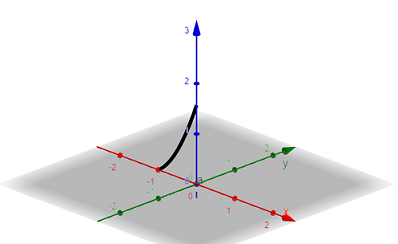# For the following curve, plot approximately its trace. Assuming that the curve gives the motion...

## Question:

For the following curve, plot approximately its trace. Assuming that the curve gives the motion of a particle, describe the motion in words.

{eq}\gamma (t)= (-1, t, t^2) {/eq}

## Graph of Parametric Curve:

Consider a parametric curve in space described by the set of equations

{eq}x=x(t) \\ y=y(t) \\ z=z(t). {/eq}

The graph of the parametric curve is found by plotting the sequence of points (x,y,z)

as the parameter t varies.

The parametric curve described by the position vector

{eq}\displaystyle \gamma(t) = (-1, t, t^2) {/eq}

is sketched in the figure below.

The curve is a parabola in the plane {eq}x=-1. {/eq}